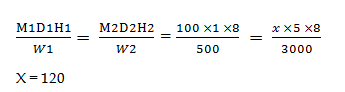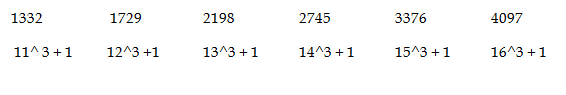# SSC CGL 2018 Practice Test Papers | Quantitative Aptitude (Day-58)

Dear Aspirants, Here we have given the Important SSC CGL Exam 2018 Practice Test Papers. Candidates those who are preparing for SSC CGL 2018 can practice these questions to get more confidence to Crack SSC CGL 2018 Examination.

[WpProQuiz 3716]

Click “Start Quiz” to attend these Questions and view Explanation

1) Simplify the given data

4562 + 3420 – 4235 + 2316 – 1237 =?

a) 4652

b) 5675

c) 4826

d) 3451

2) 1/3 of 4/5 of 15 /8 of a number is 150. Find the number

a) 300

b) 350

c) 530

d) 235

3) A seller announced a discount of 20% for stock clearance sale in that way he has to earn 10% profit in his entire sale. If the marked price of the apparel items is Rs.528 each, then what is the cost price of each apparel item?

a) 500

b) 384

c) 356

d) 600

4) A can do a piece of work in 8 days and B can do the same job in half of the efficiency of A. In how many days they both can together to finish the same job?

a) 5 days

b) 5 1/ 2 days

c) 5 1/3 days

d) 6 days

5) Find the area of square whose side is equals to length of the rectangle with the ratio of length and breadth is 6: 5. Perimeter of rectangle is 44.

a) 169

b) 196

c) 144

d) 125

6) In a Park, an average visitor of week days is 280 and an average visitor of week end is 350. What is the average visitor of a week?

a) 330

b) 350

c) 325

d) 300

7) A student got 445 marks in an examination out of 500. His mark is what percent of that total mark?

a) 90

b) 89

c) 97

d) 85

8) A Manufacture company makes 500 toys made by 100 workers in a day working 8 hours. If the company received an order of 3000 toys which is delivered in 5 days then how many more workers need to finish the order in time?

a) 10

b) 12

c) 15

d) 20

9) Find the next term of the given series

1332, 1729, 2198, 2745, 3376 , ?

a) 4094

b) 8979

c) 4097

d) 4567

10) Find the value of unit digit

11 × 12 × 13 × ………. × 19

a) 5

b) 1

c) 8

d) 0

4562 + 3420 – 4235 + 2316 – 1237

4562 + 3420 + 2316 – 4235 – 1237 = 10298  – 5472 = 4826

(1/ 3 × 4 /5 × 15 / 8) x = 150

x/2 = 150

x = 300

Marked Price = 528

Selling Price = 528 * 80/100 = 422.4

Selling price = 422.4

Cost price = 422.4 * 100 /110 =384

A – 1/ 8 days

B – 1/ 16 days

A + B = 1/ 8 + 1/ 16 = 3 / 16

A and B can finish the work in 16/ 3 i .e, 5 1/ 3 days

Perimeter of rectangle = 44

2(6x+5x) = 44

22x = 44

x = 2

Length = 12 cm, Breadth = 10 cm

Area of Square = (12)2 = 144 cm2

Total visitor in weeks days = 280 * 5 = 1400

Total visitors in weekends = 350 * 2 = 700

Total visitors in a week = 1400 + 700 = 2100

Average visitors in a week = 2100 / 7 = 300

445/500 * 100 = 89%Already we have 100 workers. So 120 – 100 = 20 more workers needed.11 × 12 × 13 × 14 × 15 × 16 × 17 × 18 × 19

Unit digits of those numbers = 1×2×3×4×5×6×7×8×9 = 0